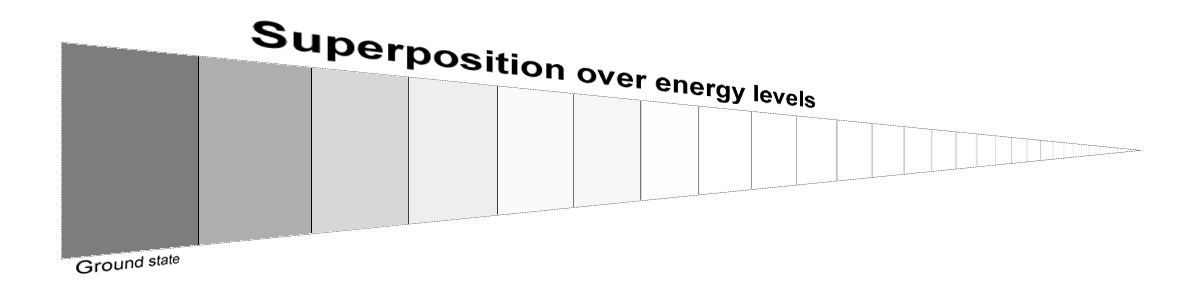# Solution: How change arises in QM

Previously I pointed out that if you drew out the wave function of the entire universe by separating out its different energy components and shading each according to its amplitude, you would find that the universe appears completely static.This is correct according to standard quantum mechanics. If you looked at how much amplitude the universe had in any particular energy level, you would find that this amplitude was not changing in size.

The only change you would observe would be in the direction, or phase, of the amplitude in the complex plane. And directions of amplitudes in the complex plane are unphysical. Right?

No! While there is an important sense in which the direction of an amplitude is unphysical (the universe ultimately only computes magnitudes of amplitudes), there is a much much more important sense in which the direction of an amplitude contains loads of physical information.

This is because when the universe is in a superposition of different energy states, the amplitudes of these states can interfere.

It is here that we can find the answer to the question I posed in the previous post. Physical changes come from interference between the amplitudes of all the energy states that the universe is in superposition over.

One consequence of all of this is that if the universe did happen to be in a pure energy state, and not in a superposition of multiple energy levels, then change would be impossible.

From which we can conclude: The universe is in a superposition of energy levels, not in any clearly defined single energy level! (Proof: Look around and notice that stuff is happening)

This doesn’t mean, by the way, that the universe is actually in one of the energy levels and we just don’t know which. It also doesn’t mean that the universe is in some other distinct state found by averaging over all of the different energy states. “Superposition” is one of these funny words in quantum mechanics that doesn’t have an analogue in natural language. The best we can say is that the universe really truly is in all of the states in the superposition at once, and the degree to which it is in any particular state is the amplitude of that state.

***

Let’s imagine a simple toy universe with one dimension of space and one of time.

This universe is initially in an equal superposition of two pure energy states Φ0(x) and Φ1(x), each of which is a real function (no imaginary components). The first has zero energy, and we choose our units so that the second has an energy level equal to exactly 1.

So the wave function of our universe at time zero can be written Ψ = Φ0 + Φ1. (I’m ignoring normalization factors because they aren’t really crucial to the point here)

And from this we can conclude that our probability density is:

P(x) = Ψ*·Ψ = Φ02 + Φ12 + 2·Φ0·Φ1

Now we advance forward in time. Applying the Schrodinger equation, we find:

Φ0(x, t) = Φ0(x)
Φ1(x, t) = Φ1(x) · e-it

Notice that both of these energy states have a time-independent magnitude. The first one is obvious – it’s just completely static. The second one you can visualize as a function spinning in the complex plane, going from purely real and positive to purely imaginary to purely real and negative, et cetera. The magnitude of the function is just what you’d get by spinning it back to its positive real value.

From our two energy functions, we can find the total wave function of the universe:

Ψ(x, t) = Φ0(x) + Φ1(x) · e-it

Already we can see that our time-dependent wave function is not a simple product of our time-independent wave function and a phase.

We can see the consequences of this by calculating the time-dependent probability density:

P(x, t) = Φ0(x)2 + Φ1(x)2 + Φ0(x) · Φ1(x) · (e-it + eit)

Or…

P(x, t) = |Φ0|2 + |Φ1|2 + 2 · Φ0(x) · Φ1(x) · cos(t)

And in our final result, we can see a clear time dependence of the spatial probability distribution over the universe. The last term will grow and shrink, oscillating over time and giving rise to dynamics.

***

We can visualize what’s going on here by looking at the time evolution of each pure energy state as if it’s spinning in the complex plane. For instance, if the universe was in a superposition of the lowest four energy levels we would see something like:The length of the arrow represents the amplitude of that energy level – “how much” the universe is in that energy state. The arrows are spinning in the complex plane with a speed proportional to the energy level they represent.

The wave function of the universe is represented by the sum of all of these arrows, as if you stacked each on the head of the previous. And this sum is changing!

For instance, in the universe’s first moment, the superposition looks like this:And later the universe looks like this:If we plotted out the first two energy states scaled by their amplitudes, we might see the following spatial distributions, initially and finally:

Even though there have been no changes in the magnitudes of the arrows (the degree to which the universe exists in each energy level) we get a very different looking universe.

This is the basic idea that explains all change in the universe, from the rising and falling of civilizations to the births and deaths of black holes: they are results of the complex patterns of interference produced by spinning amplitudes.